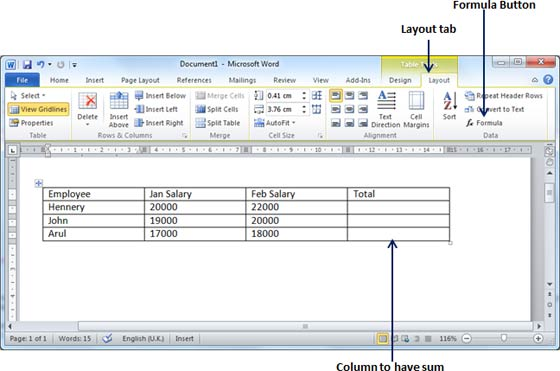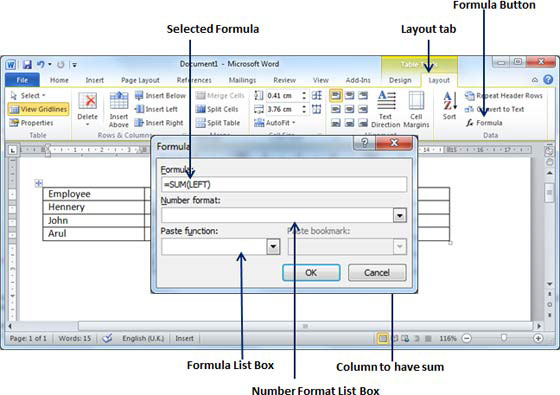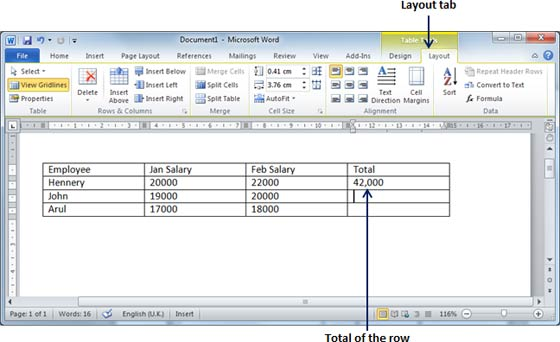# Planet For Application Life Development Presents MY IT WorldExplore and uptodate your technology skills...

Word - Add Formula

Microsoft Word allows you to use mathematical formula in table cells which can be used to add numbers, to find average of numbers, or find the largest or smallest number in table cells you specify. There is a long list of formulas from which, a formula can be used based on the requirement. This chapter will teach you how to use formula in word tables.

# Add a Formula:

Following are the simple steps to add formula in a table cell available in word document.

Step (1): Consider the following table where we will have total of the rows. Click in a cell that should contain the sum of a rows.Step (2): Now click the Layout tab and then click Formula button which will display a Formula Dialog Box which will suggest a default formula, which is =SUM(LEFT) in our case. You can select a number format using Number Format List Box to display the result or you can change the formula using Formula List Box.Step (3): Now click OK to apply the formula and you will see that left cells have been added and its sum has been put in the total cell where we wanted to have it. You can repeat the procedure to have sum of other two rows as well.# Cell Formulas:

The Formula dialog box provides following important functions to be used as formula in a cell.

FormulaDescription
AVERAGE( )The average of a list of cells.
COUNT( )The number of items in a list of cells
MAX( )The largest value in a list of cells
MIN( )The smallest value in a list of cells
PRODUCT( )The multiplication of a list of cells
SUM( )The sum of a list of cells

If you are bit familiar with spreadsheet program, you can construct your word cell formula. Word formulas use a reference system to refer to individual table cells. Each column is identified by a letter, starting with A for the first column, B for the second column, and so on. After the letter comes the row number. Thus, the first cell in the first row is A1, the third cell in the fourth row is C4, and so on.

Following are usful points to help you in constructing word cell formula.

SNCell References and Description
1A single cell reference, such as B3 or F7
2A range of cells, such as A4:A9 or C5:C13
3A series of individual cells, such as A3,B4,C5
4ABOVE, referring to all cells in the column above the current cell.
5BELOW, referring to all cells in the column below the current cell.
6LEFT, referring to all cells in the row to the left of the current cell
7RIGHT, referring to all cells in the row to the right of the current cell

You can also construct simple math expressions, such as B3+B5*10 by using simple mathematical operators +, -, /, *, %.